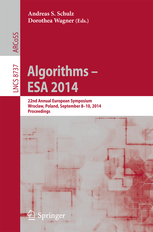• A
• A
• A
• ABC
• ABC
• ABC
• ąÉ
• ąÉ
• ąÉ
• ąÉ
• ąÉ
Regular version of the site

## Sublinear Space Algorithms for the Longest Common Substring Problem

P. 605-617.
Starikovskaya T., Vildhoj H. W., Kociumaka T.
Given $m$ documents of total length $n$, we consider the problem of finding a longest string common to at least $d \geq 2$ of the documents. This problem is known as the \emph{longest common substring (LCS) problem} and has a classic $\Oh(n)$ space and $\Oh(n)$ time solution (Weiner [FOCS'73], Hui [CPM'92]). However, the use of linear space is impractical in many applications. In this paper we show that for any trade-off parameter $1 \leq \tau \leq n$, the LCS problem can be solved in $\Oh(\tau)$ space and $\Oh(n^2/\tau)$ time, thus providing the first smooth deterministic time-space trade-off from constant to linear space. The result uses a new and very simple algorithm, which computes a $\tau$-additive approximation to the LCS in $\Oh(n^2/\tau)$ time and $\Oh(1)$ space. We also show a time-space trade-off lower bound for deterministic branching programs, which implies that any deterministic RAM algorithm solving the LCS problem on documents from a sufficiently large alphabet in $\Oh(\tau)$ space must use $\Omega(n\sqrt{\log(n/(\tau\log n))/\log\log(n/(\tau\log n)})$ time.



### In bookVol. 8737. Berlin: Springer, 2014.